#### Initial conditions

As stated at the beginning of the chapter, the initial conditions provide one of the three general sources of information regarding the state. Therefore, three alternative types of initial conditions will be allowed:

1. Known State: The initial state,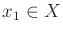, is given. This initializes the problem with perfect state information. Assuming nature actions interfere with the state transition function,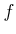, uncertainty in the current state will generally develop.
2. Nondeterministic: A set of states,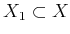, is given. In this case, the initial state is only known to lie within a particular subset of. This can be considered as a generalization of the first type, which only allowed singleton subsets.
3. Probabilistic: A probability distribution,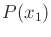, overis given.
In general, let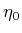denote the initial condition, which may be any one of the three alternative types.

Steven M LaValle 2020-08-14